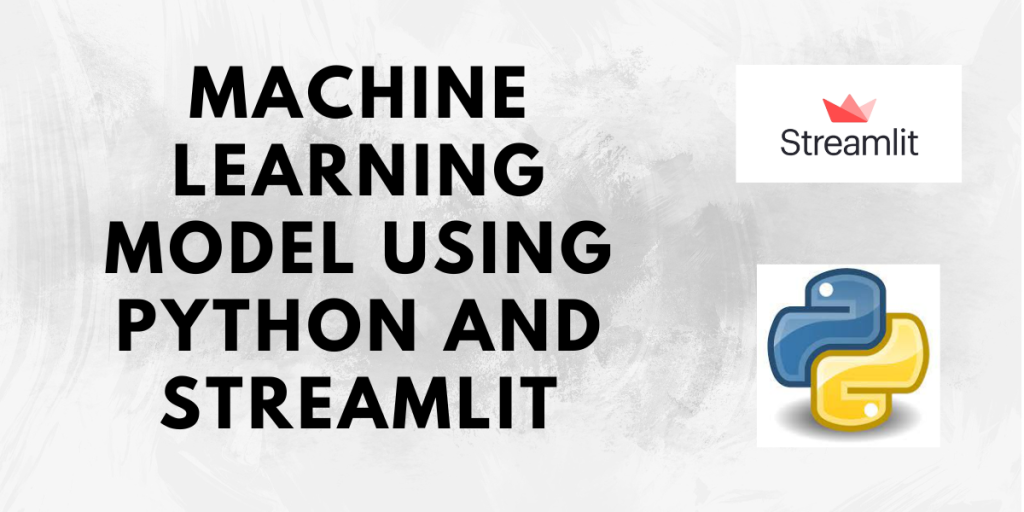# Deploying a Machine Learning Model using Streamlit – House Price Prediction GUIHello and welcome to this tutorial! Since you have stumbled upon this tutorial, we guess you are performing some machine-learning task at hand. Often while working in machine learning, we use Jupyter Notebook, Google Collab, or any of our favorite IDE. But there is no use of the model trained in these IDEs for someone who is not familiar with them.

To solve this problem, we need to create an easily usable application so that everyone can use the machine learning model. In this tutorial, we will first create a simple Linear Regression model and then deploy it using Streamlit.

Before beginning, please make sure you go through the below tutorial on Streamlit as we will be using the text elements and widgets covered in it while developing the application.

## Setting up the Project Structure

The project structure for the predictive model will be like this:

We will need three Python scripts, `app.py` for the web app, `houseprice_model.py` for developing the machine learning model and `predict_cost.py` for predicting the price of the house. We will also need a `data` folder to store the dataset.

## Developing the machine learning model

We will be using a House Price dataset here. It consists of 5 columns viz.

• Area of the house
• Number of bedrooms in the house
• Number of balconies
• Age of the house i.e. how old the house is
• Price of the house

The dataset looks like this:

Remember that this dataset contains arbitrary values for all the parameters in it.

Since building a Linear regression model is beyond the scope of this article, we will not be getting into the details of it. Feel free to check out the Linear Regression from Scratch tutorial to learn about Linear regression and its Python implementation.
Below is the entire code for our machine-learning model.

We will use the houseprice_model.py file for writing the code for the machine-learning model.

houseprice.py

```# Importing the required libraries
import numpy as np
import pandas as pd
from sklearn.model_selection import train_test_split
from sklearn.linear_model import LinearRegression

# Seperating the target and features
# target ->y, features -> X
y = data['price']
X = data.drop(columns='price', axis=1)

# Splitting into training and test set
X_train, X_test, y_train, y_test = train_test_split(X, y, test_size=0.3, random_state=1)

# Making the model
lr = LinearRegression()
lr.fit(X_train, y_train)

# Predicting the output
y_pred = lr.predict(X_test)

# Saving the model
import joblib

joblib.dump(lr, "lr_model.sav")
```

In the end, after building the model we saved it using the `joblib` module as `lr_model.sav` in order to use it later.

## Designing the frontend with Streamlit

If you don’t have Streamlit installed yet, you can install using the command

```pip install streamlit
```

Now, as we saw earlier, we will use the file `app.py` for the app. So we will code the front end in this file.

Since we have 4 independent features, area, number of bedrooms, number of balconies and age of the house, we will require 4 input fields to get the values of these features. And since we want to predict the price of the house, we will use a button, which when clicked upon will display the price of the house.

app.py

```import streamlit as st
from predict_cost import predict
import numpy as np

st.title('Home price prediction')

st.write('---')

# area of the house
area = st.slider('Area of the house', 1000, 5000, 1500)

# no. of bedrooms in the house
bedrooms = st.number_input('No. of bedrooms', min_value=0, step=1)

# no. of balconies in the house
balconies = st.radio('No. of balconies', (0, 1, 2 , 3))

# how old is the house? (age)
age = st.number_input('How old is the house (in years)?', min_value=0, step=1)

if st.button('Predict House Price'):
cost = predict(np.array([[area, bedrooms, balconies, age]]))
st.text(cost)
```

We can run the app using the command

```streamlit run app.py
```

In the above code, all the parameter limits are arbitrary. Also, we have imported the `predict` function from the predict_cost.py file. When the Predict House Price button is clicked the predict function is called.

predict_cost.py

```import joblib

def predict(data):
Here, in the `predict` function, we use the joblib module to load the model that we had saved and then return the predicted price based on the data sent to the function.
While passing the data to the `predict` function, we need to pass it as a 2-d array. Hence, we have converted the input data to a NumPy array. Also, the predict function returns a 1-d array, so while printing the value we have written cost to get the sole value in the returned array.